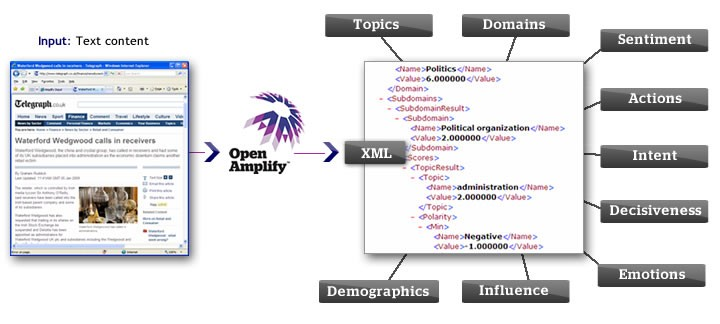# Text Mining - term frequency – inverse document frequency (tf-idf)

tf–idf, short for term frequency–inverse document frequency, is a numerical statistic that is intended to reflect how important a word is to a document in a collection or corpus.

It is often used as a weighting factor in information retrieval and text mining.

The tf-idf value increases proportionally to the number of times a word appears in the document, but is offset by the frequency of the word in the corpus, which helps to control for the fact that some words are generally more common than others.

## Type of Weight

### TF

TF rewards tokens that appear many times in the same document. If a word occurs often in a document, then it is more important to the meaning of the document.

TF is computed as the frequency of a token in a document (bag of words)

Example:

• a document d contains 100 tokens
• the token t appears in d 5 times,

$$\text{TF weight (of the token in the document)} = \frac\text{Number of times the token appears in the document}}{\text{Total Number of Tokens in the document}} = \frac{5}{100} = \frac{1}{20}$$

### IDF

IDF rewards tokens that are rare overall in a dataset. If a rare word occurs in two documents, then it is more important to the meaning of each document.

IDF weight for a token, t, in a set of documents, U, is computed as follows:

$$IDF(t) = \frac{\text{Total number of documents}}{\text{Number of documents in U that contain t}} = \frac{N}{n(t)}$$

Note that $\frac{n(t)}{N}$ is the frequency of t in U, and $\frac{N}{n(t)}$ is the inverse frequency.

### TF-IDF

Finally, to bring it all together, the total TF-IDF weight for a token in a document is the product of its tf and idf weights.

$$\text{TF-IDF} = TF \times IDF$$

## Scope of Weight

### Local

Sometimes token weights depend on the document the token belongs to, that is, the same token may have a different weight when it's found in different documents. We call these weights local weights. TF is an example of a local weight, because it depends on the length of the source.

### Global

On the other hand, some token weights only depend on the token, and are the same everywhere that token is found. We call these weights global, and IDF is one such weight.

## Calculation

### IDF

Python with Spark:

rdd = sc.parallelize([('Doc1', ['Hello','nico','MonPoussin']), ('Doc2', ['Hello','Toi']),  ('Doc3', ['Hello','Pfff','nico'])])
N = rdd.count()
(rdd.flatMap(lambda (x,y):list(set(y)))
.map(lambda x: (x,1.0))
.reduceByKey(lambda a,b:a+b)
.map(lambda (x,y):(x,N/y))
.collect())

[('MonPoussin', 3.0),
('Toi', 3.0),
('nico', 1.5),
('Pfff', 3.0),
('Hello', 1.0)]


## Documentation / Reference

Recommended Pages(Natural|Human) Language - Text (Mining|Analytics)

See Tweet Web site comments Weblogs Forum comment ... A tweet is analyzed differently than a long blog post and a blog comment is analyzed differently than a tweet. If you want to use any...NLP - Term-document Matrix

A term-document matrix is an important representation for text analytics. Each row of the matrix is a document vector, with one column for every term in the entire corpus. Naturally, some documents...Process - Poisson Process

The Poisson process is a stochastic process in which events occur: continuously independently (of the time since the last event) - (ie random) at a constant / known average rate in a fixed interval...Text Mining - Bag of (words|tokens)

The idea is to treat strings (documents), as unordered collections of words, or tokens, i.e., as bags of words. Bag of words techniques all apply to any sort of token, a “bag-of-words” is then much...